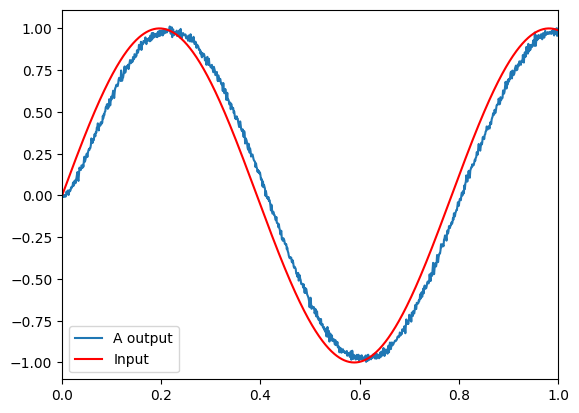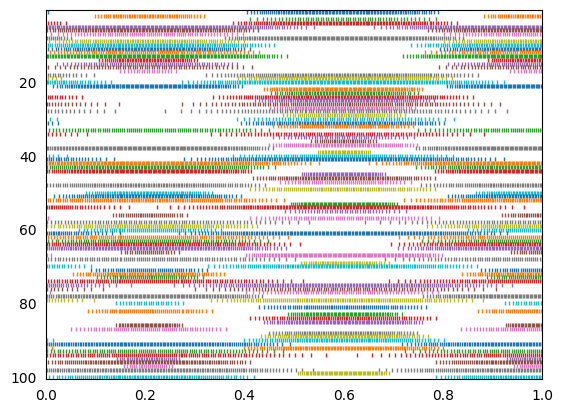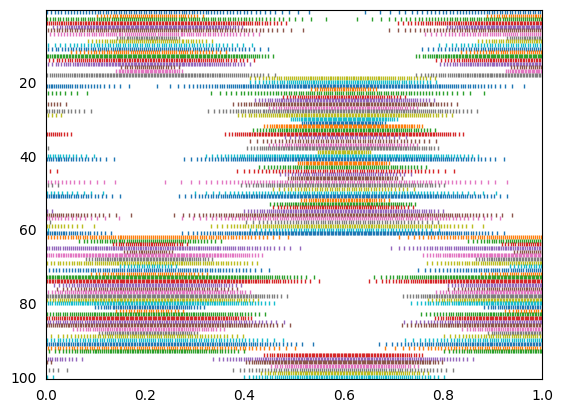Note

This documentation is for a development version. Click here for the latest stable release (v4.0.0).

# Many neurons¶

This demo shows how to construct and manipulate a population of neurons.

These are 100 leaky integrate-and-fire (LIF) neurons. The neuron tuning properties have been randomly selected.

The input is a sine wave to show the effects of increasing or decreasing input. As a population, these neurons do a good job of representing a single scalar value. This can be seen by the fact that the input graph and neurons graphs match well.

:

%matplotlib inline
import matplotlib.pyplot as plt
import numpy as np

import nengo
from nengo.utils.ensemble import sorted_neurons
from nengo.utils.matplotlib import rasterplot


## Step 1: Create the neural population¶

Our model consists of a single population of neurons.

:

model = nengo.Network(label="Many Neurons")
with model:
# Our ensemble consists of 100 leaky integrate-and-fire neurons,
# representing a one-dimensional signal
A = nengo.Ensemble(100, dimensions=1)


## Step 2: Create input for the model¶

We will use a sine wave as a continuously changing input.

:

with model:
sin = nengo.Node(lambda t: np.sin(8 * t))  # Input is a sine


## Step 3: Connect the network elements¶

:

with model:
# Connect the input to the population
nengo.Connection(sin, A, synapse=0.01)  # 10ms filter


## Step 4: Probe outputs¶

Anything that is probed will collect the data it produces over time, allowing us to analyze and visualize it later.

:

with model:
sin_probe = nengo.Probe(sin)
A_probe = nengo.Probe(A, synapse=0.01)  # 10ms filter
A_spikes = nengo.Probe(A.neurons)  # Collect the spikes


## Step 5: Run the model¶

:

# Create our simulator
with nengo.Simulator(model) as sim:
# Run it for 1 second
sim.run(1)


## Step 6: Plot the results¶

:

# Plot the decoded output of the ensemble
plt.figure()
plt.plot(sim.trange(), sim.data[A_probe], label="A output")
plt.plot(sim.trange(), sim.data[sin_probe], "r", label="Input")
plt.xlim(0, 1)
plt.legend()

# Plot the spiking output of the ensemble
plt.figure()
rasterplot(sim.trange(), sim.data[A_spikes])
plt.xlim(0, 1)

:

(0.0, 1.0)The top graph shows the decoded response of the neural spiking. The bottom plot shows the spike raster coming out of every 2nd neuron.

:

# For interest's sake, you can also sort by encoder
indices = sorted_neurons(A, sim, iterations=250)
plt.figure()
rasterplot(sim.trange(), sim.data[A_spikes][:, indices])
plt.xlim(0, 1)

:

(0.0, 1.0)